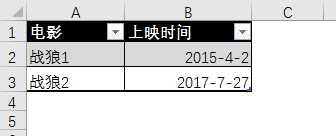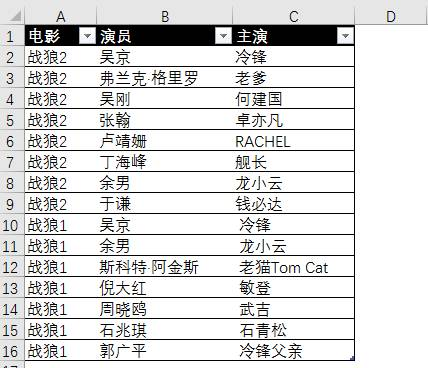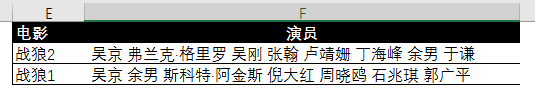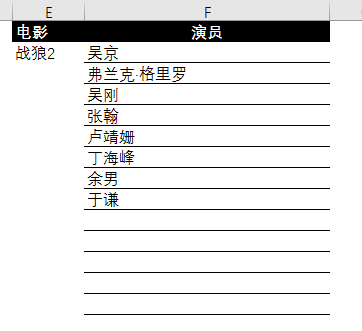# 战狼2中的Excel问题，你会几个？“哦！”老祝松了口气，不清不淡的应了声。

“我爱余男也爱卢靖姗。”老祝摆出一副抢答的欠揍的模样儿。=DATEDIF(B2,B3,”y”)

=DATEDIF(B2,B3,”m”)

=TEXT(SUM(DATEDIF(B2,B3,{“y”,”ym”,”md”})*10^{4,2,0}),”00年00月00天”)

02年03月25天。”=VLOOKUP(“吴京”,B:C,2,0)

=INDEX(B:B,MATCH(“冷锋”,C:C,))

=SUMPRODUCT(1/COUNTIF(B2:B16,B2:B16))”F2单元格输入公式，向下复制填充：

=SUBSTITUTE(PHONETIC(OFFSET(A\$1:B\$1,MATCH(E2,A:A,)-1,,COUNTIF(A:A,E2))),E2,” “)F2单元格输入数组公式：

{=INDEX(B:B,SMALL((A\$1:A\$16<>E\$2)/1%+ROW(\$1:\$16),ROW(A1)))&””}